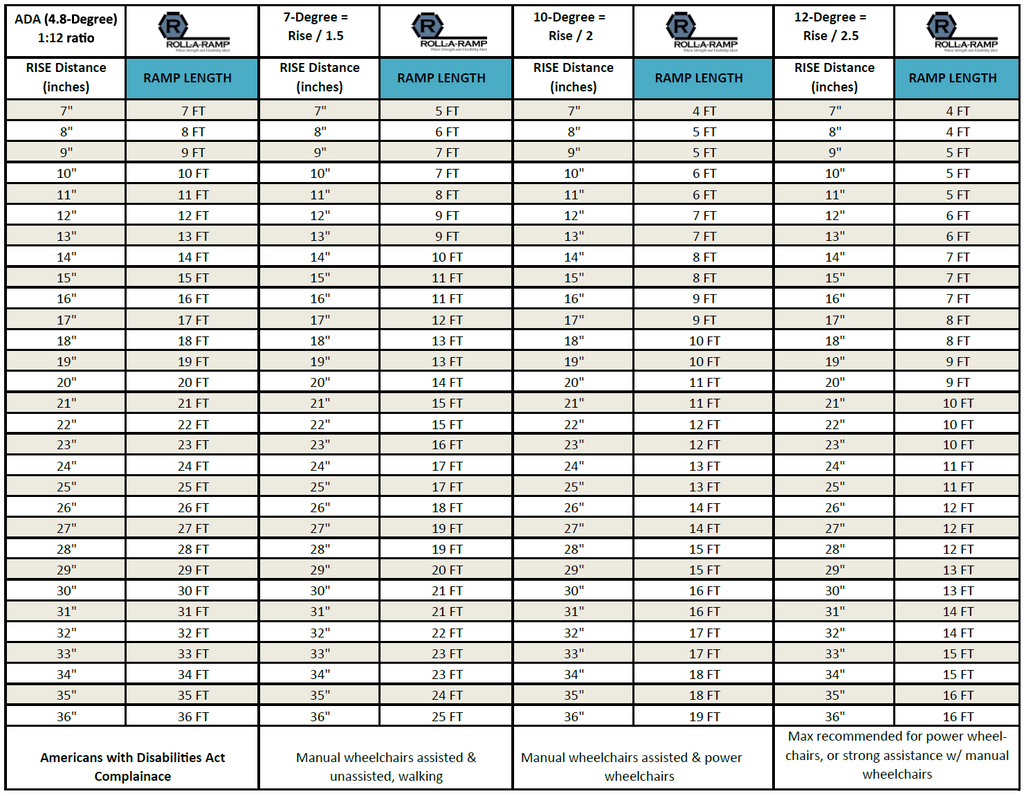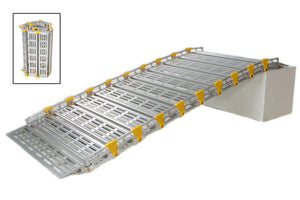Questions? Call us at 1-855-HRZN-MID 📞 (1-855-479-6643) | M-F 9a-6p ET
Questions? Call us at 1-855-HRZN-MID

# Ramp Length Calculator - What Ramp Length Do I Need?

## What length do I need?

The length you need depends on the application and how much slope (how steep the angle) is acceptable to you. The guide will show how public use, ADA compliance, personal use, power chair, manual wheelchair all affect how much ramp you need.

### General Rules

1. Measure vertical height of steps or landing in inches.
2. Divide by 2 to get length needed in feet.
• Example: 24 inch height divided by 2 equals 12. So 12 feet of ramp needed.### Length determined by use:

• Public Use: ADA compliance requires a 1:12 slope ( or 1 foot of ramp for every 1 inch of vertical rise).
• Walking, Manual Wheelchairs, Power chairs, Scooters: Vertical rise divided by 1.5. (Example: 24" rise divided by 1.5 = 16' ramp length)
• Walking, Manual Wheelchair with able-bodied helper, Power chairs, scooters: Vertical rise divided by 2. (Example: 24" rise divided by 2 = 12' ramp length)

### ﻿Ramp Length Calculator

Courtesy of Roll-A-Ramp.